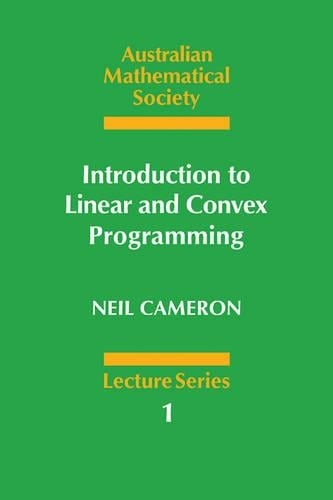•# Introduction to Linear and Convex Programming - Australian Mathematical Society Lecture Series (Paperback)

(author)
£29.99
Paperback 160 Pages / Published: 05/09/1985
• We can order this from the publisher

Usually dispatched within 15 working days

This introduction to optimization emphasizes the need for both a pure and an applied mathematical point of view. Beginning with a chapter on linear algebra and Euclidean geometry, the author then applies this theory with an introduction to linear programming. There follows a discussion of convex analysis, which finds application in non-linear programming. The book ends with an extensive commentary to the exercises that are given at the end of each chapter. The author's straightforward, geometrical approach makes this an attractive textbook for undergraduate students of mathematics, engineering, operations research and economics.

Publisher: Cambridge University Press
ISBN: 9780521312073
Number of pages: 160
Weight: 250 g
Dimensions: 228 x 152 x 15 mm